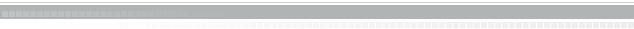KTH MathematicsSF2972 Game Theory, 7.5 creditsGame theory provides mathematical tools for the analysis of strategic interactions, with applications to many fields, ranging from political science and economics to biology and computer science. The goal of this course is to give a mathematically rigorous introduction to some of the classical game-theoretic models and solution concepts.

### Old exams

To Mathematical Statistics
To Mathematical Statistics Courses